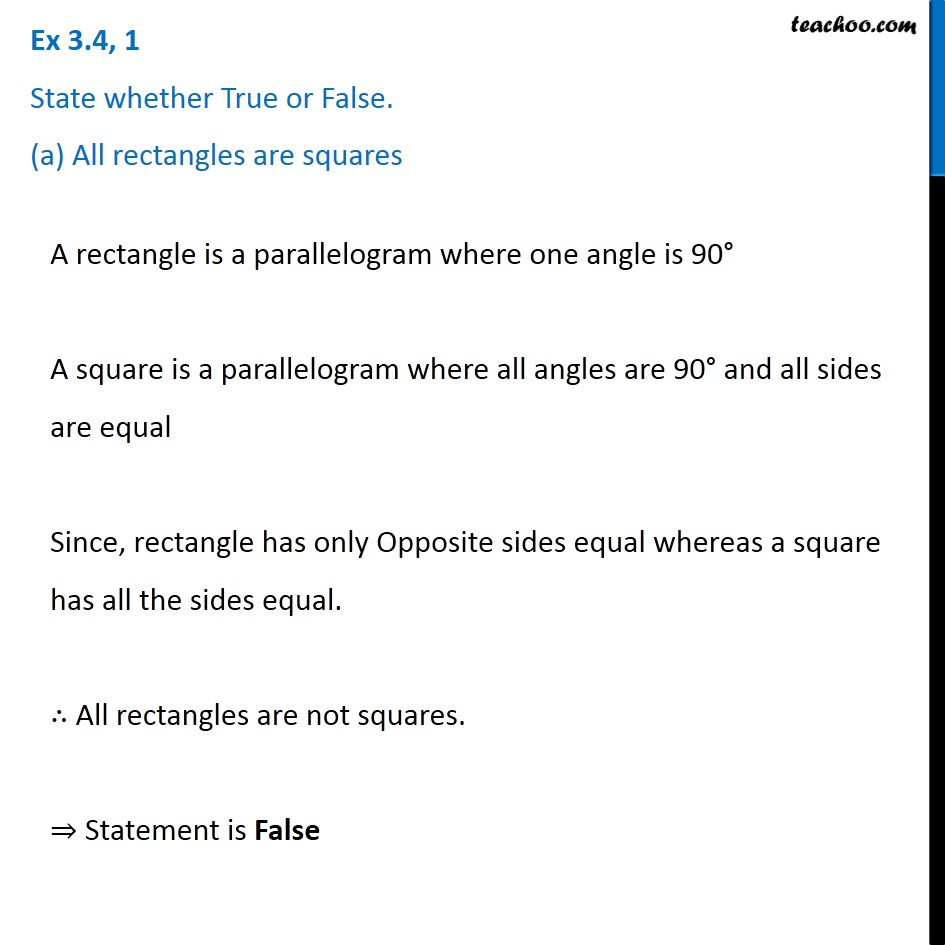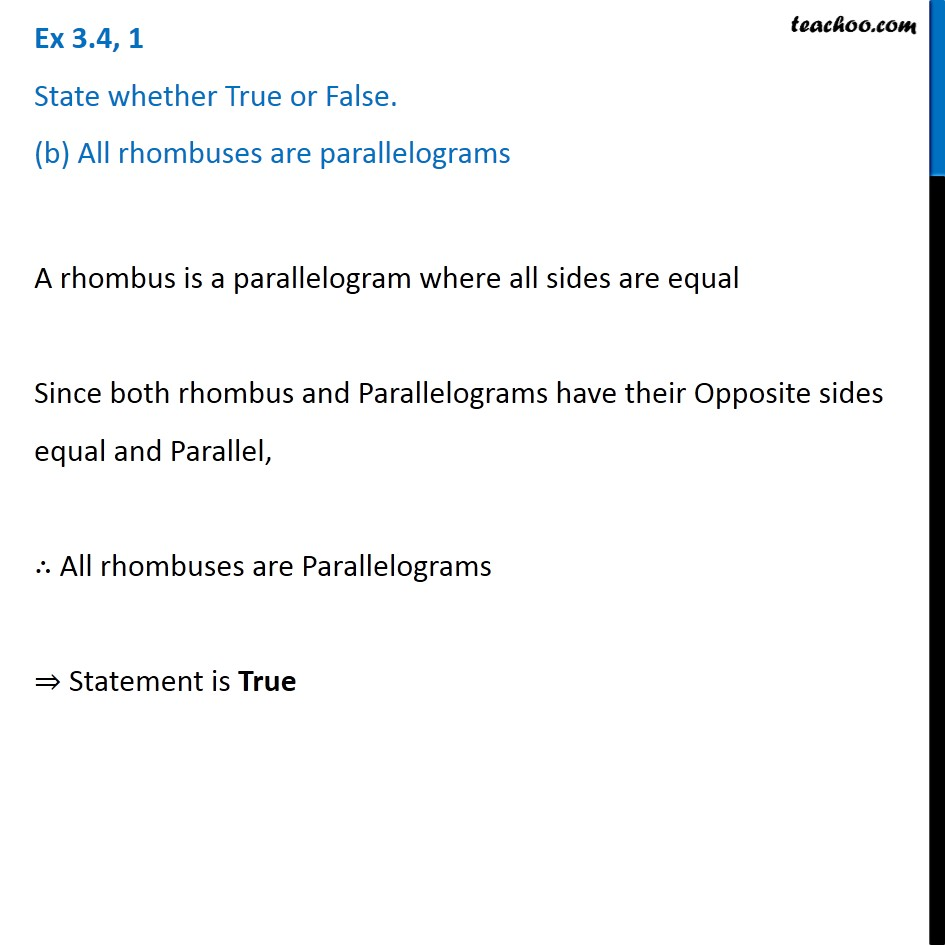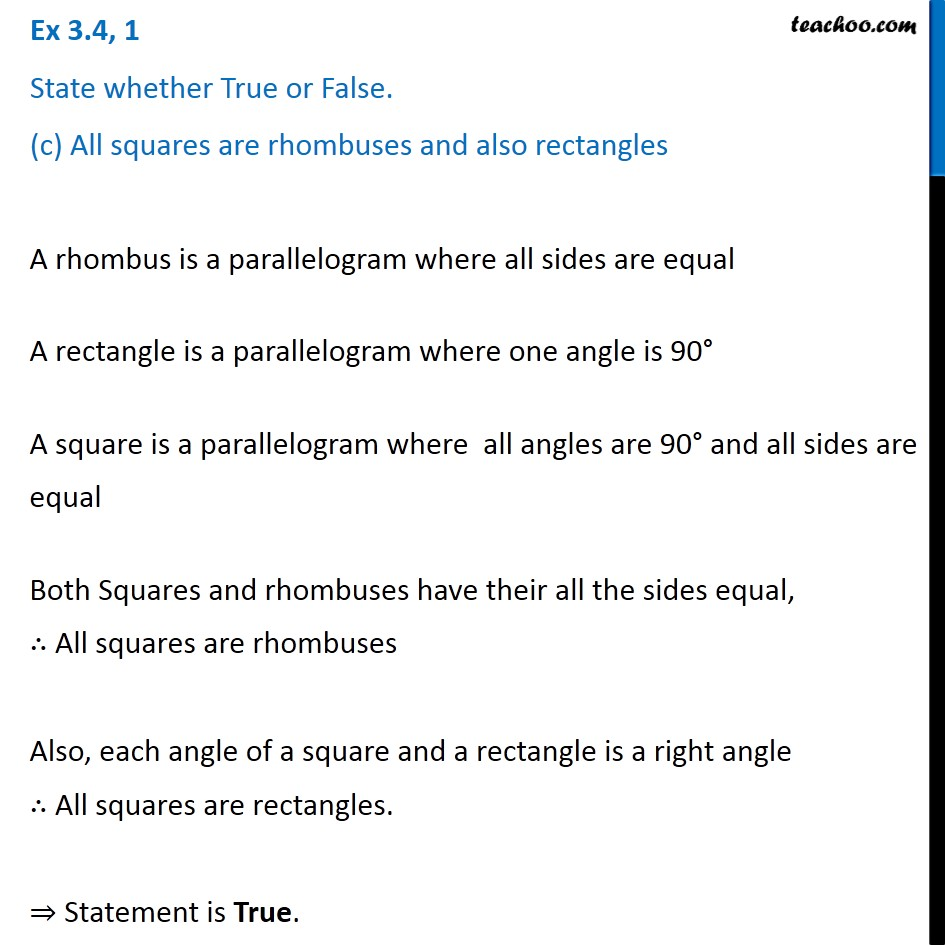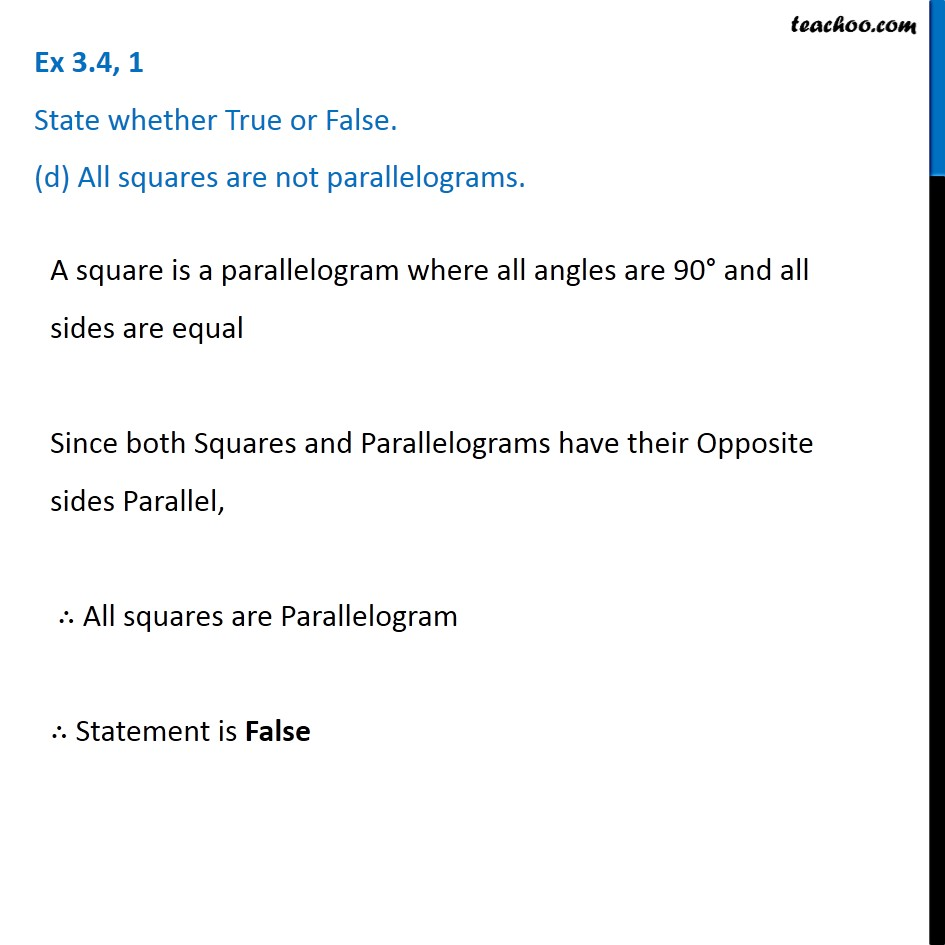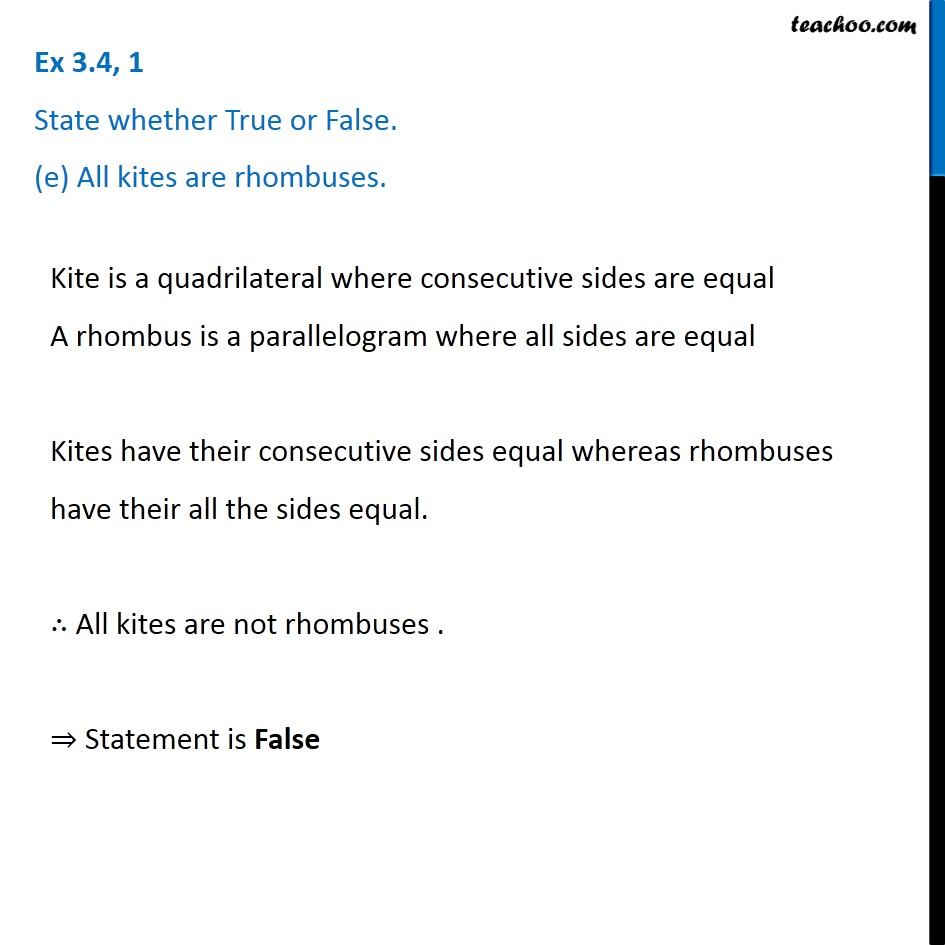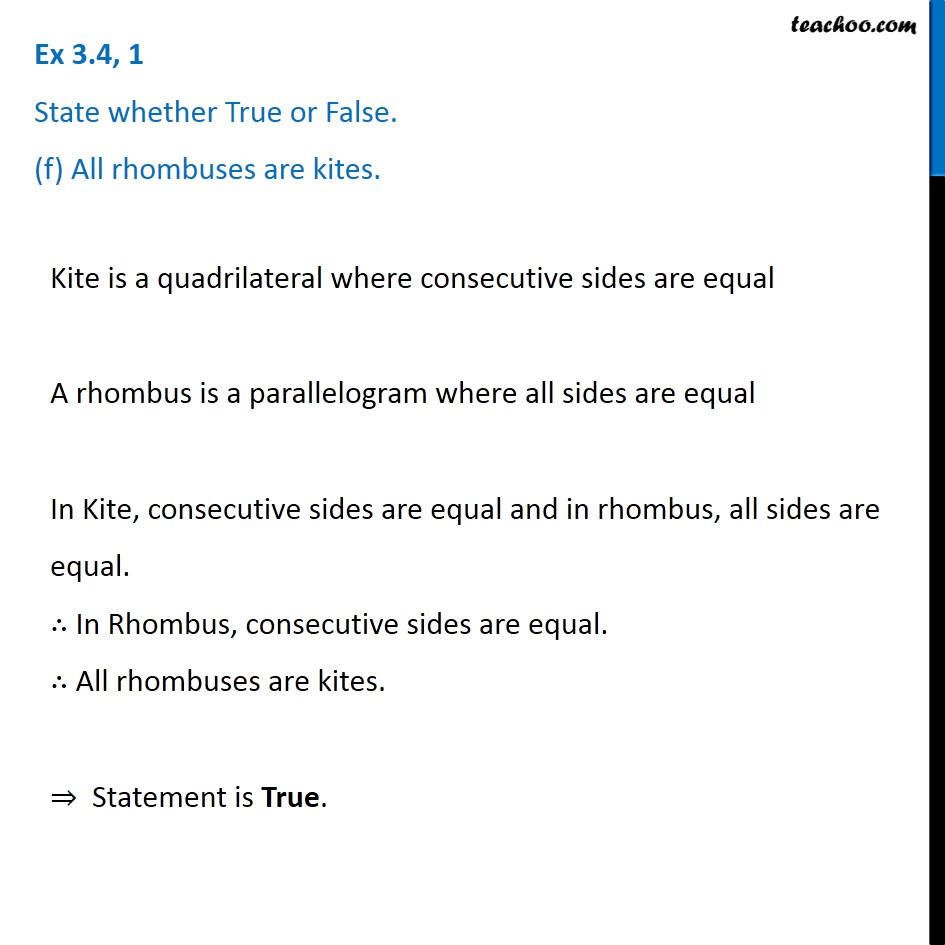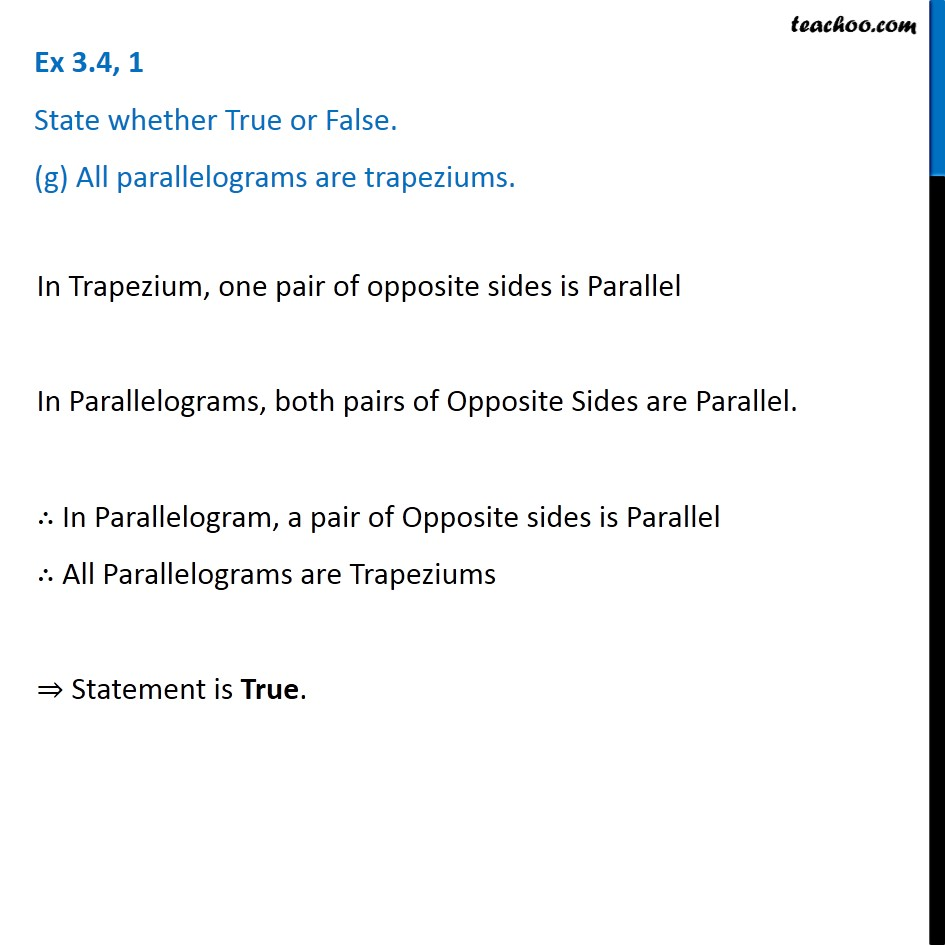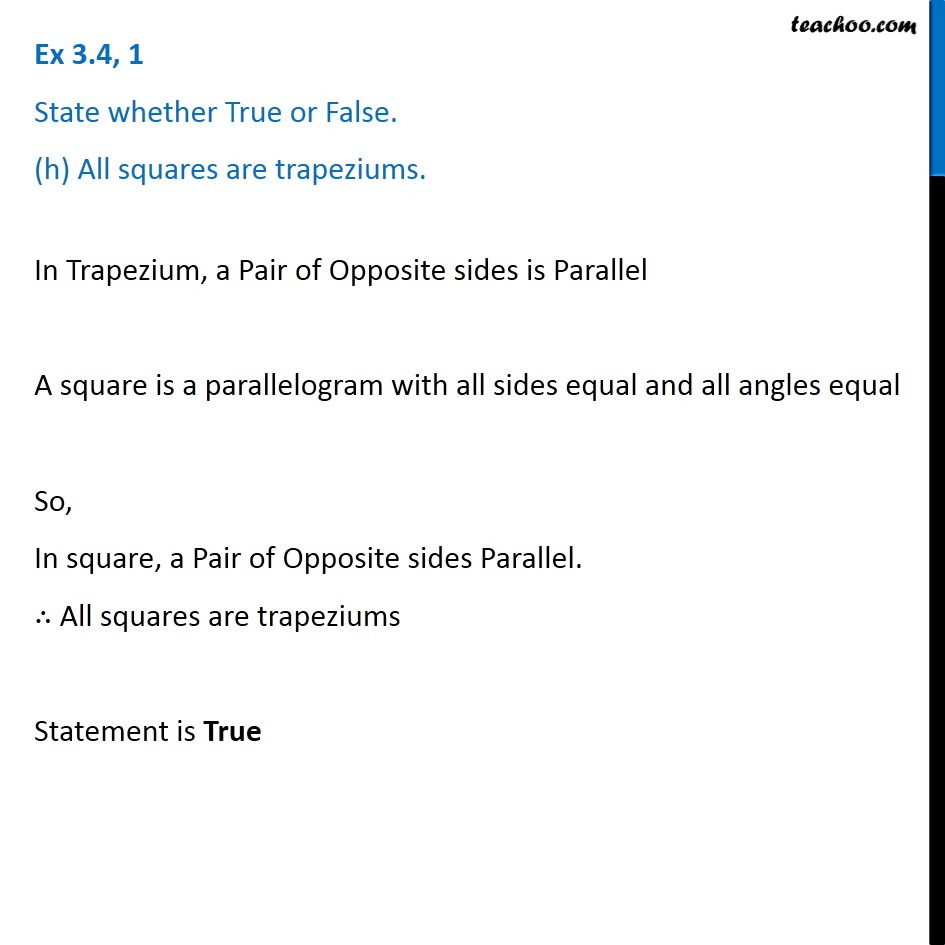Subscribe to our Youtube Channel - https://you.tube/teachoo

1. Chapter 3 Class 8 Understanding Quadrilaterals
2. Concept wise
3. Rhombus, Rectangle, Square

Transcript

Ex 3.4, 1 State whether True or False. (a) All rectangles are squares A rectangle is a parallelogram where one angle is 90° A square is a parallelogram where all angles are 90° and all sides are equal Since, rectangle has only Opposite sides equal whereas a square has all the sides equal. ∴ All rectangles are not squares. ⇒ Statement is False Ex 3.4, 1 State whether True or False. (b) All rhombuses are parallelograms A rhombus is a parallelogram where all sides are equal Since both rhombus and Parallelograms have their Opposite sides equal and Parallel, ∴ All rhombuses are Parallelograms ⇒ Statement is True Ex 3.4, 1 State whether True or False. (c) All squares are rhombuses and also rectangles A rhombus is a parallelogram where all sides are equal A rectangle is a parallelogram where one angle is 90° A square is a parallelogram where all angles are 90° and all sides are equal Both Squares and rhombuses have their all the sides equal, ∴ All squares are rhombuses Also, each angle of a square and a rectangle is a right angle ∴ All squares are rectangles. ⇒ Statement is True. Ex 3.4, 1 State whether True or False. (d) All squares are not parallelograms. A square is a parallelogram where all angles are 90° and all sides are equal Since both Squares and Parallelograms have their Opposite sides Parallel, ∴ All squares are Parallelogram ∴ Statement is False Ex 3.4, 1 State whether True or False. (e) All kites are rhombuses. Kite is a quadrilateral where consecutive sides are equal A rhombus is a parallelogram where all sides are equal Kites have their consecutive sides equal whereas rhombuses have their all the sides equal. ∴ All kites are not rhombuses . ⇒ Statement is False Ex 3.4, 1 State whether True or False. (f) All rhombuses are kites. Kite is a quadrilateral where consecutive sides are equal A rhombus is a parallelogram where all sides are equal In Kite, consecutive sides are equal and in rhombus, all sides are equal. ∴ In Rhombus, consecutive sides are equal. ∴ All rhombuses are kites. ⇒ Statement is True. Ex 3.4, 1 State whether True or False. (g) All parallelograms are trapeziums. In Trapezium, one pair of opposite sides is Parallel In Parallelograms, both pairs of Opposite Sides are Parallel. ∴ In Parallelogram, a pair of Opposite sides is Parallel ∴ All Parallelograms are Trapeziums ⇒ Statement is True. Ex 3.4, 1 State whether True or False. (h) All squares are trapeziums. In Trapezium, a Pair of Opposite sides is Parallel A square is a parallelogram with all sides equal and all angles equal So, In square, a Pair of Opposite sides Parallel. ∴ All squares are trapeziums Statement is True

Rhombus, Rectangle, Square